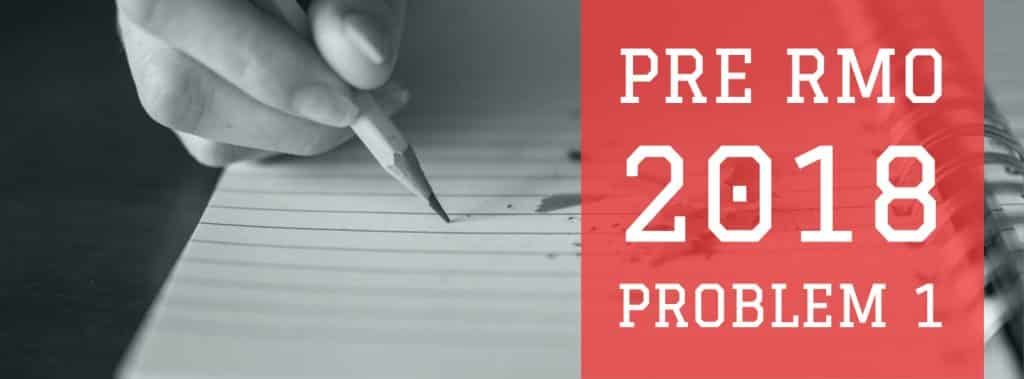How Cheenta works to ensure student success?
Explore the Back-Story

# Prime factor of last page - Pre RMO 2018 Problem 1 DiscussionA book is published in three volumes, the pages being numbered from 1 onwards. The page numbers are continued from the first volume to the third. The number of pages in the second volume is 50 more than in the first volume, and the number pages in the third volume is one and a half times that in the second. The sum of the page numbers on the first pages of the three volumes is $1709$. If $n$ is the last page number. What is the largest prime factor of $n$?

Hint 1:

Suppose the number of pages is the First Volume is t. It's first page is numbered 1. Then the second volume has t+50 pages. Its first page is numbered t+1. Finally, the third volume has $\frac{3}{2} \times ( t + 50)$ pages. Its first page is (t + t + 50 + 1 =) 2t + 51.

Hint 2 :

Sum of the page numbers of first pages of three volumes is: 1 + t +1 + 2t + 51 = 3t + 51. 3t + 51 = 1709 This implies t= 552 Hint 3 The total number of pages in three volumes is $t + t + 50 + \frac{3}{2} \times (t+50) \\ = \frac {4t + 100 + 3t + 150}{2} \\ = \frac { 7 \times 552 + 250}{2} \\ = 2057 = 11^2 \times 17$.

Hence the greatest prime factor is $17$.

A book is published in three volumes, the pages being numbered from 1 onwards. The page numbers are continued from the first volume to the third. The number of pages in the second volume is 50 more than in the first volume, and the number pages in the third volume is one and a half times that in the second. The sum of the page numbers on the first pages of the three volumes is $1709$. If $n$ is the last page number. What is the largest prime factor of $n$?

Hint 1:

Suppose the number of pages is the First Volume is t. It's first page is numbered 1. Then the second volume has t+50 pages. Its first page is numbered t+1. Finally, the third volume has $\frac{3}{2} \times ( t + 50)$ pages. Its first page is (t + t + 50 + 1 =) 2t + 51.

Hint 2 :

Sum of the page numbers of first pages of three volumes is: 1 + t +1 + 2t + 51 = 3t + 51. 3t + 51 = 1709 This implies t= 552 Hint 3 The total number of pages in three volumes is $t + t + 50 + \frac{3}{2} \times (t+50) \\ = \frac {4t + 100 + 3t + 150}{2} \\ = \frac { 7 \times 552 + 250}{2} \\ = 2057 = 11^2 \times 17$.

Hence the greatest prime factor is $17$.

This site uses Akismet to reduce spam. Learn how your comment data is processed.

### 2 comments on “Prime factor of last page - Pre RMO 2018 Problem 1 Discussion”

1.Adithya Sajeevan says:

Didn't get the second hint that how 1 + t + 1 + 2t + 51 = 3t + 51.

Why the two 1s re not added here? Why it isn't 3t + 53?!

1.Rishabh Jain says:

It was misprint. Typing error.. It's only 3t+53

### Knowledge Partner